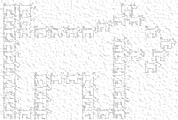# Small Chaos Incorporated## Chaos### Fractal Puppies

The main image on this page is the result of a mathematical equation. Chaotic behavior is observed by iterating certain functions repeatedly, feeding each result back into the equation. This creates the series f(x), f(f(x)), f(f(f(x))), etc.... The function used to create the Fractal Schnauzer Puppies, our corporate mascot, consists of both an x and a y component. Regardless of the initial value used for the function, iterating the function and plotting the results yields the image of a puppies composed of self-similar puppies, which in turn are composed of self-similar puppies, ad infinitum. While the points plotted move about the graph in a random chaotic fashion, as they are generated, the result is far from random. The well ordered image, called the attractor set, is produced, illustrating the bounds of the underlying chaos.About Us | Site Map | Privacy Policy | Legal Notices | Contact Us